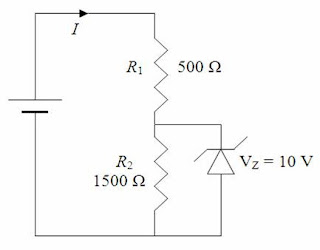## Wednesday, May 04, 2011

### Kerala Engineering Entrance (KEAM) 2011 Question (MCQ) on Zener Diode

“Everything should be made as simple as possible, but not simpler”

– Albert Einstein

The following multiple choice question on zener diode was included in Kerala Engineering Entrance (KEAM) 2011 question paper. If you identify that R1 is the current limiting resistor and R2 is the load resistor in the simple shunt voltage regulator making use of a zener diode, you won’t have any confusion. Here is the question:In the circuit given the current through the zener diode is

(a) 10 mA

(b) 6.67 mA

(c) 5 mA

(d) 3.33 mA

(e) 0 mA

Since the zener breakdown voltage (across the zener diode) is 10 V, the voltage across the resistor R2 is 10 V. The current through R2 is 10 V/1500 Ω = 0.00667 A = 6.67 mA.

The voltage drop across R1 is 15 V – 10 V = 5 V.

Therefore, the current through R1 is 5 V/500 Ω = 0.01 A = 10 mA

The above current gets divided between the resistor R2 and the zener diode. Therefore, the current through the zener diode is 10 mA – 6.67 mA = 3.33 mA.

By clicking on the label ‘zener diode’ below this post you can access similar questions on zener diodes posted on this site.

#### 1 comment:

1.I love reading your blog keep sharing! I think? I am just mesmerized.
aluminum tubing distributors